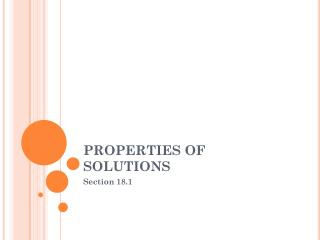DownloadDownload PresentationPROPERTIES OF SOLUTIONS

# PROPERTIES OF SOLUTIONS

Télécharger la présentation## PROPERTIES OF SOLUTIONS

- - - - - - - - - - - - - - - - - - - - - - - - - - - E N D - - - - - - - - - - - - - - - - - - - - - - - - - - -
##### Presentation Transcript

1. PROPERTIES OF SOLUTIONS Section 18.1

2. AFTER READING SECTION 18.1, YOU SHOULD KNOW: • factors that increase the rate at which a solute dissolves • the difference between an unsaturated, saturated and supersaturated solution • how to read the graphs of pages 504 & 505 • how the solubility of a gas and pressure are related

3. FACTORS THAT AFFECT THE RATE AT WHICH A SOLUTE DISSOLVES • Temperature • And increase in the kinetic energy will cause an increase in the rate that a solute dissolves • Surface Area • An increase in surface area will increase the rate at which a solute dissolves • Aggitation • Stirring or shaking will cause the solute to dissolve faster

4. SOLUBILITY • Unsaturated Solution • A solution that contains less than the maximum amount of solute for a given amount of solvent at a constant temp • Saturated Solution • A solution that contains the maximum amount of solute for a given amount of solvent at a constant temperature • Supersaturated Solution • A solution that contains more than the maximum amount of solute for a given amount of solvent at a constant temperature

5. Miscible • Two liquids that will dissolve into each other • Ex: HCl and water, milk and water • Immiscible • Two liquids that will not dissolve into each other • Ex: oil and water, hexane and water

6. SOLUBILITY AND TEMPERATURE

7. HENRY’S LAW • Gas solubility increases as the partial pressure of the gas above the solution increases S1 = S2 P1 P2 When a bottle is closed, the liquid is under high pressure, so the CO2 is dissolved into the liquid When the bottle is opened, the pressure decreases, which causes the solubility of CO2 to decreases

8. AFTER READING SECTION 18.1, YOU SHOULD KNOW: • factors that increase the rate at which a solute dissolves • the difference between an unsaturated, saturated and supersaturated solution • how to read the graphs of pages 504 & 505 • how the solubility of a gas and pressure are related